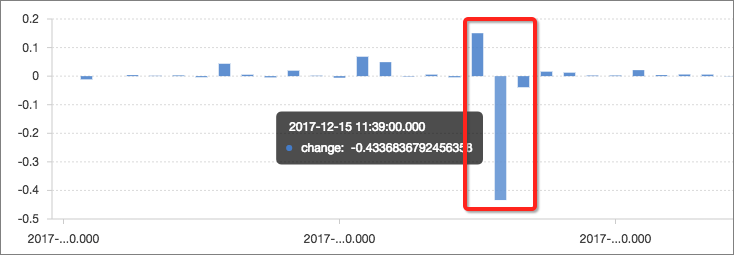The query and analysis features of Log Service follow the SQL-92 standard and support various mathematical statistics and computing methods. Currently, Log Service supports saving query statements through Saved Search. Log Service also supports setting the trigger cycle (interval) for queries, setting judgment conditions for execution results, and reporting alerts. You can set an alerting action to specify the way to inform you when the execution result of a regular saved search operation meets the trigger conditions.

## Background information

Currently, the following three notification methods are supported:
• Notification center: You can set multiple contacts in the Alibaba Cloud notification center. The system sends notifications to contacts through emails and SMS messages.
• WebHook: includes DingTalk Chatbot and custom WebHook.
• (Coming soon) Writing back to Log Service Logstores: You can subscribe to events through Realtime Compute and Function Compute, or check views and reports for alerts.
For more information about how to configure the alerting feature, see Configure an alert. In addition to the monitoring and alerting features of Log Service, you can use CloudMonitor to monitor all metrics of Log Service. CloudMonitor can send you a notification when the alerting condition is triggered.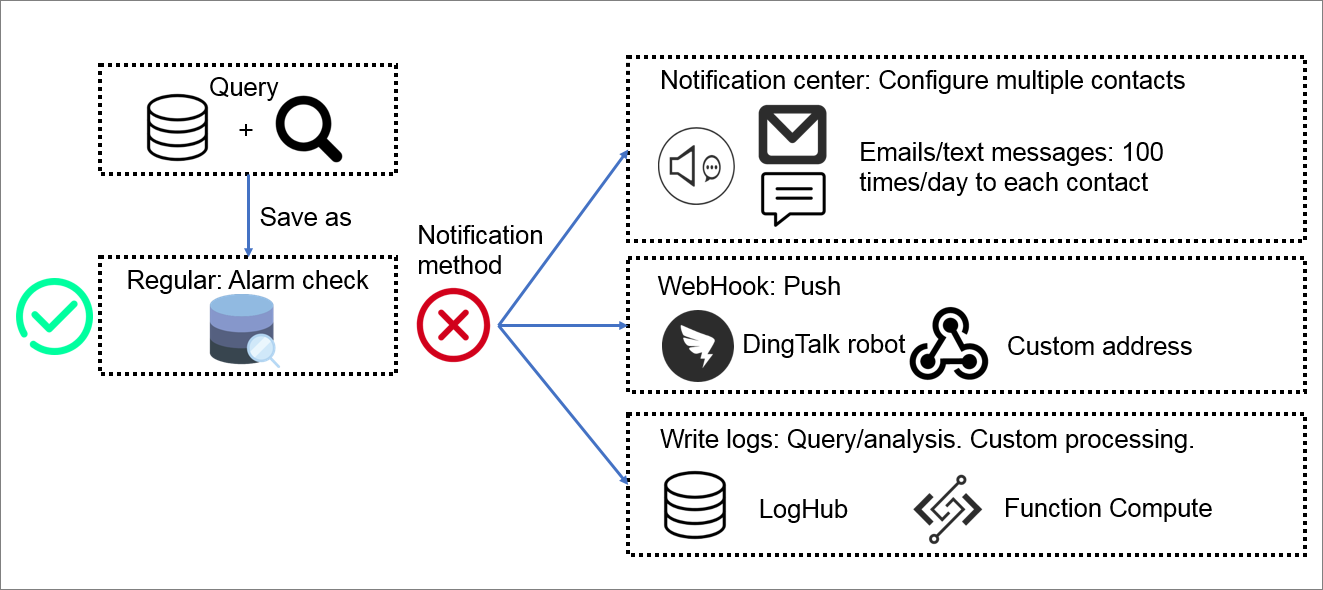## Scenario

This section takes NGINX logs as an example to describe how to query and analyze collected logs regularly through Log Service and check whether the following business issues exist based on the query result:
• Whether an error exists.
• Whether a performance issue exists.
• Whether a sudden decrease or increase of the traffic exists.

## Preparation (NGINX log access)

1. Collect log data.
1. On the Overview page, click Import Data in the upper-right corner. In the dialog box that appears, click Nginx - Text Log.
2. Select a Logstore.
If you enter the process of configuring log collection by clicking the plus sign (+) next to Data Import under a Logstore on the Logstores tab, the system skips this step.
3. Create a machine group.
Before creating a machine group, make sure that you have installed Logtail.
• ECS instances: Select an ECS instance, and click Install. ECS instances running Windows do not support one-click installation of Logtail. In this case, you need to manually install Logtail. For more information, see Install Logtail in Windows.
• User-created machines: Install Logtail as prompted. For more information about how to install Logtail, see Install Logtail in Linux or Install Logtail in Windows based on your operating system.
After installing Logtail, click Complete Installation to create a machine group. If you have created a machine group, click Use Existing Server Groups.
4. Configure the machine group.
Select a machine group and move the machine from Source Server Groups to Applied Server Groups.
5. Specify the following configuration items: Config Name, Log Path, NGINX Log Configuration, and NGINX Key. You can specify Advanced Options based on your needs.
6. Click Next.
2. Complete query and analysis configurations.
3. Set the views and alerts for key metrics.
The following figure shows sample views.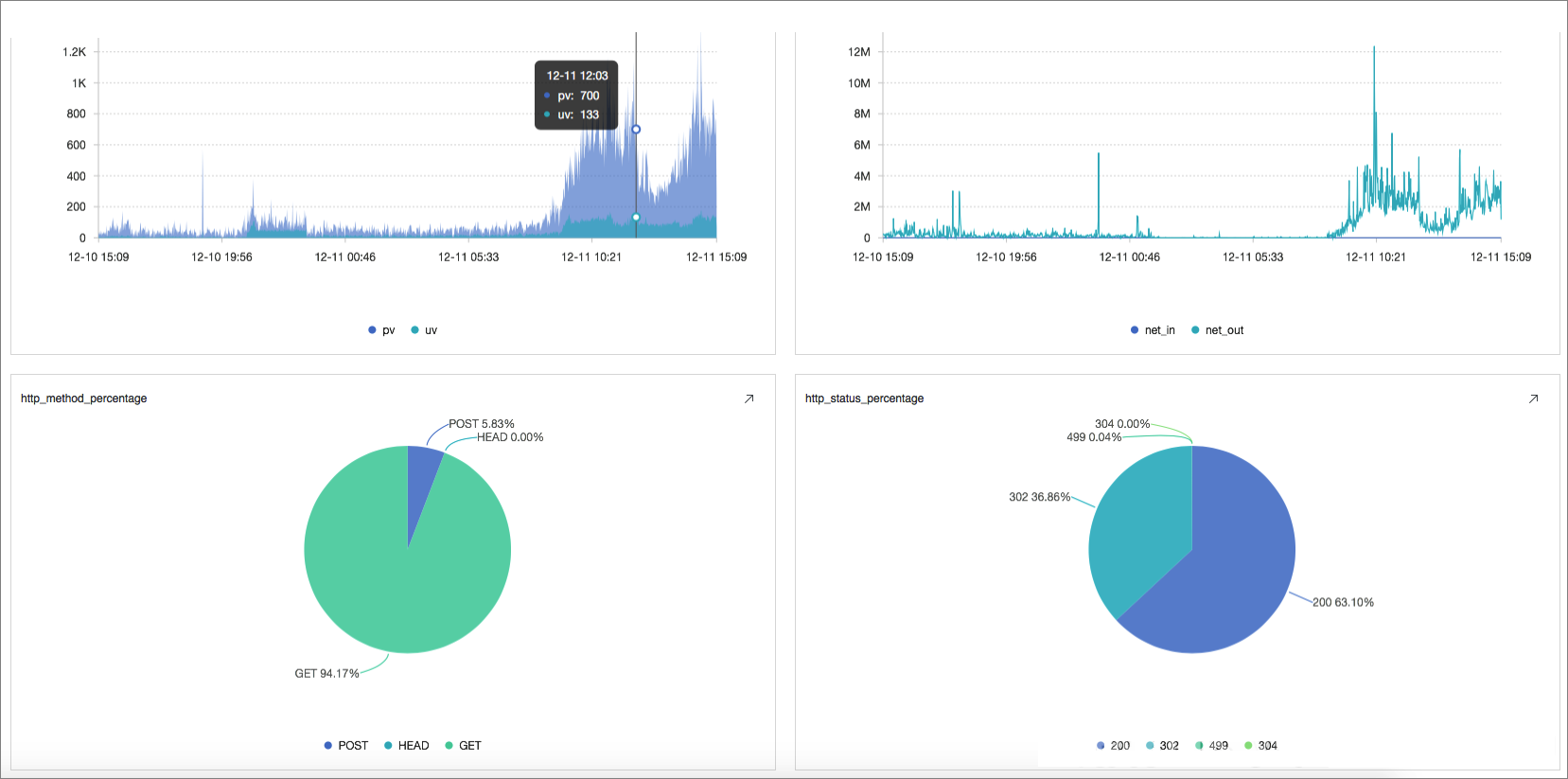## Check whether any error exists

The common error codes include 404 (the request cannot find the address), 502, and 500 (an error occurs with the server). You only need to focus on 500 errors.

To check whether a 500 error exists, you can run the following query statement to count the number of errors (c) per unit time. Then, you can set the alert rule as c > 0, indicating that an alert will be sent when the number of 500 errors exceeds 0 in the unit time.
``status:500 | select count(1) as c``

This method is simple but too sensitive. For services facing high business pressure, a few 500 errors are common. In response to this situation, you can set the trigger count to 2 in the trigger conditions so that alerts are only triggered when the conditions are met for 2 times in a row.

## Check whether any performance issue exists

Although no error occurs in server operation, the latency might be increased. You can set an alert to check the latency.

For example, you can calculate the latency of all the write requests `Post` of the operation `/adduser` by using the following method: Set the alert rule as `l > 300000`, indicating that an alert will be sent when the average latency exceeds 300 ms.
``Method:Post and URL:"/adduser" | select avg(Latency) as l``
Sending alerts based on the average latency is simple and direct. However, this method may average the latency of individual requests, making it difficult to detect issues. For example, you can compute a mathematical distribution for the latency in the time period, namely, dividing the latency into 20 intervals and calculating the number in each interval. As shown in the histogram, the latency of most requests is lower than 20 ms, but the highest latency reaches 2.5s.
``Method:Post and URL:"/adduser" | select numeric_histogram(20, Latency)``You can use the percentile in mathematical statistics (the maximum latency is 99%) as the trigger condition. This excludes false alerts triggered by accidental high latency and reflects the overall situation of the latency. The following statement calculates the latency of the 99th percentile `approx_percentile(Latency, 0.99)`. You can also modify the second parameter to calculate the latency of other percentiles, for example, the request latency of the 50th percentile `approx_percentile(Latency, 0.5)`.
``Method:Post and URL:"/adduser" | select approx_percentile(Latency, 0.99) as p99``
In a monitoring scenario, you can chart the average latency, the 50th percentile latency, and the 90th percentile latency. The following figure shows the latency of every minute in a day (1,440 minutes).
``* | select avg(Latency) as l, approx_percentile(Latency, 0.5) as p50, approx_percentile(Latency, 0.99) as p99, date_trunc('minute', time) as t group by t order by t desc limit 1440``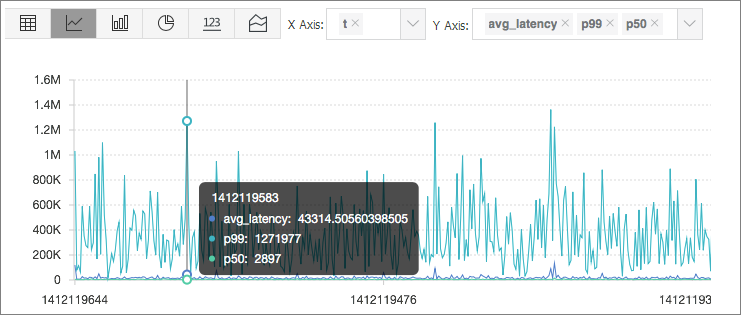## Check whether the traffic has a sudden decrease or increase

The natural traffic on the server is usually in line with probability distribution, which means that a process of slow increase or decrease exists. The sudden decrease or increase of the traffic indicates great changes in a short time period. This phenomenon is usually abnormal and needs special attention.

As shown in the following monitoring chart, the traffic decreases by over 30% within 2 minutes and resumes rapidly within 2 minutes.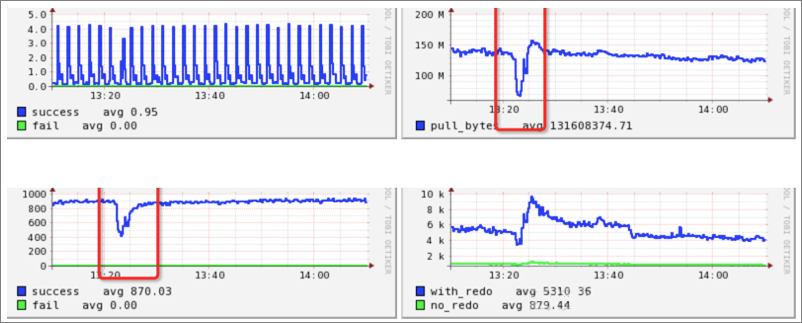The following reference frames are provided for a sudden decrease or increase:
• Last window: compares data in the current time period with that in the previous time period.
• Window of the same time period of the previous day: compares data in the current time period with that in the same time period of the previous day.
• Window of the same time period of the previous week: compares data in the current time period with that in the same time period of the previous week.
This section takes the first reference frame as an example to calculate the change ratio of inbound traffic. You can also calculate other metrics of the traffic such as queries per second (QPS).
1. Define a calculation window.
Define a window of 1 minute to calculate the inbound traffic size of this minute. The following figure shows the statistic result within a 5-minute interval.
``* | select sum(inflow)/(max(__time__)-min(__time__)) as inflow , __time__-__time__%60  as window_time from log group by window_time order by window_time limit 15``
As shown in the result, the average inbound traffic size specified by `sum(inflow)/(max(__time__)-min(__time__))` in every window is even.2. Calculate the difference in the window (max_ratio).
Subqueries are involved. Run a query statement to calculate the change ratio between the maximum value or the minimum value and the average value from the preceding result. In this example, the change ratio between the maximum value and the average value is calculated, for example, 1.02. You can set the alert rule as max_ratio > 1.5, indicating that an alert will be sent when the ratio of change exceeds 50%.
`` * | select max(inflow)/avg(inflow) as max_ratio from (select sum(inflow)/(max(__time__)-min(__time__)) as inflow , __time__-__time__%60  as window_time from log group by window_time order by window_time limit 15) ``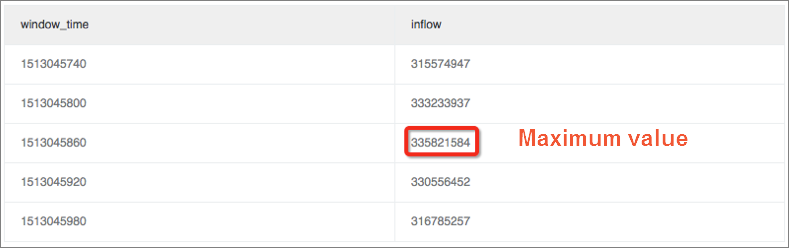3. Calculate the difference in the window (latest_ratio).
In some scenarios, more attention is paid to the fluctuation of the latest value (whether the value is recovered). You can use the max_by function to get the inbound traffic size of the latest window (specified by windows_time). Then, you can calculate the change ratio between the latest value and the average value, for example, 0.97.
`` * | select max_by(inflow, window_time)/1.0/avg(inflow) as lastest_ratio from (select sum(inflow)/(max(__time__)-min(__time__)) as inflow , __time__-__time__%60  as window_time from log group by window_time order by window_time limit 15) ``
Note The calculation result of the max_by function is of the character type, which must be converted to the numeric type. To calculate the relative ratio of changes, you can replace the clause following SELECT with `(1.0-max_by(inflow, window_time)/1.0/avg(inflow)) as lastest_ratio`.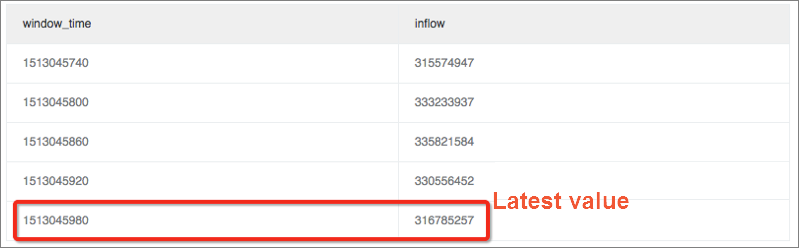4. Calculate the difference in the window which indicates the fluctuation ratio, namely, the change ratio between the current value and the previous value.
Another method for calculating the fluctuation ratio is the first derivative in mathematics, namely, the change ratio between the value of the current window and the value of the previous window.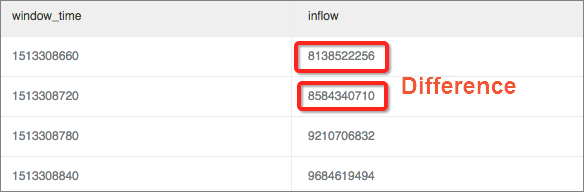Use the window function (lag) for calculation. Extract the current inbound traffic and the inbound traffic of the previous window to calculate the difference by using `inflow "lag(inflow, 1, inflow)over() "` and divide the calculated difference value by the current value to get the change ratio.
`` * | select (inflow- lag(inflow, 1, inflow)over() )*1.0/inflow as diff, from_unixtime(window_time) from (select sum(inflow)/(max(__time__)-min(__time__)) as inflow , __time__-__time__%60  as window_time from log group by window_time order by window_time limit 15) ``

In this example, a relatively major decrease occurs in traffic at 11:39, with a change ratio of over 40%.

To define an absolute change ratio, you can use the abs function for calculating the absolute value to unify the calculation result.